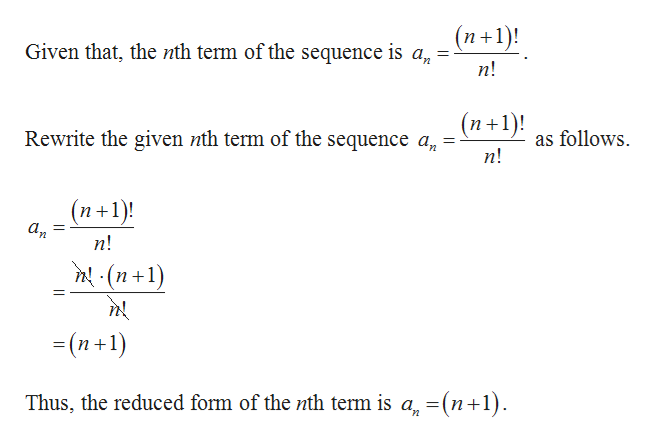# For Exercise, write the first five terms of the sequence.an=(n+1)!/n!

Question
2 views

For Exercise, write the first five terms of the sequence.

an=(n+1)!/n!

check_circle

Step 1help_outlineImage Transcriptionclose(n+1)! Given that, the nth term of the sequence is a, =- п! (n+1)! Rewrite the given nth term of the sequence a, = n! as follows. (n+1)! п! n! (n+1) - (п+1) Thus, the reduced form of the nth term is a, =(n+1). fullscreen
Step 2help_outlineImage TranscriptioncloseSubstitute n=1 in a, =(n+1), a = (1+1) Substitute n= 2 in a, =(n+1), a, = (2+1) = 3 Substitute n= 3 in a, =(n+1), az =(3+1) = 4 Substitute n= 4 in a, =(n+1), a̟ = (4 +1) fullscreen

### Want to see the full answer?

See Solution

#### Want to see this answer and more?

Solutions are written by subject experts who are available 24/7. Questions are typically answered within 1 hour.*

See Solution
*Response times may vary by subject and question.
Tagged in

### Other